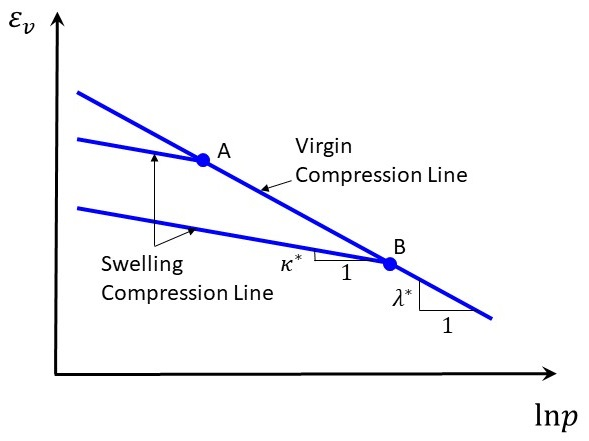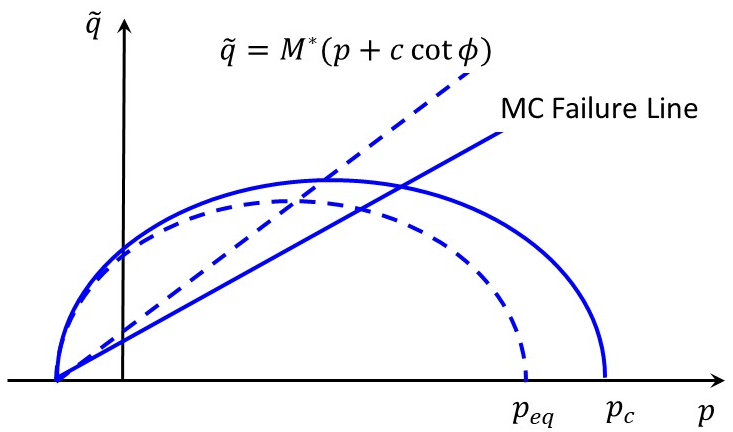FLAC3D Theory and Background • Constitutive Models

# Soft-Soil Model*

Note

*This model is available in FLAC3D only.

Soft soils generally refer to normally consolidated or slightly over-consolidated clays, silty-clays, clayey silts, and peats. Significant compression (e.g., $$E^{ref}_{oed} < 0.5 E^{ref}_{50}$$) is one of the main engineering characteristics of the soft soils. The soft-soil (SS) model has the following features: (a) pressure-dependent moduli; (b) unloading-reloading distinct from the virgin loading; (c) expansion of the volumetric yield ellipse-shaped cap; and (d) conventional Mohr-Coulomb shear failure and tension failure criteria.

In the SS model, the virgin compression assumes the relation

(1)$\Delta \epsilon_v = - \frac{\lambda^* \Delta p}{p+c\cot{\phi}}$

where $$\Delta \epsilon_v$$ is the increment of the volumetric strain, $$\Delta p$$ is the increment of the mean pressure, and material parameters $$c$$ and $$\phi$$ are the cohesion and friction angle of the soil, respectively. The material parameter $$\lambda^*$$ is the modified compression index.

(2)$\Delta \epsilon^e_v = - \frac{\kappa^* \Delta p}{p+c\cot{\phi}}$

where $$\Delta \epsilon^e_v$$ is the increment of the elastic volumetric strain, and the material parameter $$\kappa^*$$ is the modified swelling index. Equation (2) implies that the elastic bulk modulus is pressure-dependent and with the form

(3)$K = \frac{p+c\cot{\phi}}{\kappa^*}$

It is noted that the material parameters $$\lambda^*$$ and $$\kappa^*$$ (using the superscript asterisk to denote “modified”) are based on the increment of volumetric strains (Figure 1); they are different from the material parameters $$\lambda$$ and $$\kappa$$ used in the modified Cam-Clay model which are based on the increment of void ratios.Figure 1: Relation between the volumetric strain $$\epsilon_v^p$$ and the effective mean pressure $$p$$.

The model assumes a constant Poisson’s ratio $$\nu$$ , so the elastic shear modulus is

(4)$G = \frac{3(1-2\nu)}{2(1+\nu)} K$

The pre-consolidation pressure, or cap pressure, $$p_c$$, is the largest pressure level experienced by the soil. During the virgin (primary) loading, $$p_c$$ increases with the stress level and causes the plastic (irreversible) volumetric strains. By Equations (1) and (2) together with $$\Delta \epsilon_v = \Delta \epsilon^e_v + \Delta \epsilon^p_v$$, it can be derived that

(5)$\Delta p_c = -p_c \frac{\Delta \epsilon^p_v}{\lambda^* - \kappa^*}$

The yield surface of the SS model is an ellipse in the $$(p,\tilde{q})$$ plane (Figure 1) expressed as

(6)$f = p_{eq} - p_c$

where the equivalent pressure is defined as

(7)$p_{eq} = p + \frac{\tilde{q}^2}{M^2(p+c\cot{\phi})}$

and $$p=-(\sigma_1+\sigma_2+\sigma_3)/3$$, $$\tilde{q}=-[\sigma_1+(\delta-1)\sigma_2-\delta\sigma_3]$$, where $$\sigma_1$$, $$\sigma_2$$, and $$\sigma_3$$ are effective principal stress with the order $$\sigma_1 \leq \sigma_2 \leq \sigma_3$$, $$\delta=(3+\sin{\phi})/(3-\sin{\phi})$$, and $$M$$ determines the ratio of the radius of the ellipse defined by the yield function as Equation (6). Here $$M$$ is different from the value of $$6\sin{\phi}/(3-\sin{\phi})$$ in the modified Cam-Clay model. Instead, $$M$$ is not an input material parameter but internally determined by other material parameters by the relation (Brinkgreve, 1994)

(8)$M = 3 \sqrt{\frac{(1-K_{nc})^2}{(1+2K_{nc})^2} + \frac{(1-K_{nc})(1-2\nu)(\lambda^*-\kappa^*)}{(1+2K_{nc})(1-2\nu)\lambda^* - (1-K_{nc})(1+\nu)\kappa^*}}$

where $$K_{nc}$$ is the coefficient of lateral earth pressure in a normally consolidated condition, and the user can input a value or its default value is $$K_{nc} = 1 - \sin{\phi}$$ if not assigned.Figure 2: Yield and failure surfaces of the Soft-Soil model in the $$(p, \tilde{q})$$ plane.

The SS model uses the associated flow rule for the volumetric evolution, so the potential function $$g$$ for volumetric plasticity is the same as the yield function in Equation (6)

(9)$g = f = p_{eq} - p_c$

The conventional Mohr-Coulomb shear failure and tension failure criteria are also adopted in the SS model. The elastic region is defined by the volumetric yield ellipse, the Mohr-Coulomb shear failure line, and the tension failure line in the $$(p,\tilde{q})$$ plane.

If the creep effect of the secondary compression would be taken into account, the Soft-Soil-Creep model is recommended.

References

Brinkgreve, R.B.J. Geomaterial models and numerical analysis of softening. Ph.D. Thesis. Univ., Delft, Netherlands (1994).

soft-soil Model Properties

Use the following keywords with the zone property command to set these properties of the Soft-Soil model.

soft-soil
friction f

friction angle, $$\phi$$, The default value is 30 [in degrees]. The recommended input value is the critical-state friction angle rather than a higher value at small strains. Input of a zero value is not allowed.

kappa-modified f

modified swelling index, or slope of elastic swelling line, $$\kappa^*=\kappa/(1+e)$$, where $$\kappa$$ is the swelling index in the Cam-Clay model, and $$e$$ can be approximately the initial or average void ratio during a swelling path; or $$\kappa^* \approx [(1+2K_0)(1-\nu)/(1+\nu)] C_s/[(\ln{10})(1+e)]$$, where $$C_s$$ is the 1-D swelling index, $$\nu$$ is the Poisson’s ratio and $$K_0$$ is the at-rest earth pressure coefficient. Typical value of $$C_s$$ is $$C_s \approx (1/5) \sim (1/10) C_c$$. A good estimation is $$C_s \approx PI(\%)/370$$.

lambda-modified f

modified compression index, or slope of elastic compression line, $$\lambda^*=\lambda/(1+e)$$, where $$\lambda$$ is the compression index in the Cam-Clay model, and $$e$$ can be approximately the initial or average void ratio during a compression path; or $$\lambda^*=C_c/[(\ln{10})(1+e)]$$, where $$C_c$$ is the 1-D compression index. Typical value of $$C_c$$ can be estimated (Terzaphi & Peck 1967) as $$C_c=0.009(LL-100)$$ for normally consolidated clay where $$LL$$ is the liquid limit. Based on the modified Cam-Clay model, it can estimated that $$C_c \approx PI(\%)/74$$, where $$PI$$ is the plastic index.

poisson f

Poisson’s ratio, $$\nu$$, The default value is 0.15.

stress-1-effective f

initial minimum effective principal stress, $$\sigma^0_1$$. Only for calculation of the initial moduli, will not be update once assigned.

stress-2-e f

initial median effective principal stress, $$\sigma^0_2$$. Only for calculation of the initial moduli, will not be update once assigned.

stress-3-effective f

initial maximum effective principal stress, $$\sigma^0_3$$. Only for calculation of the initial moduli, will not be update once assigned.

coefficient-normally-consolidation f (a)

normal consolidation coefficient, $$K_{nc}$$. It is not allowed to be less than $$\nu / (1-\nu)$$, a range 0.5 to 0.7 is common. The default is $$K_{nc} = 1 - \sin \phi$$.

cohesion f (a)

effective cohesion, $$c$$. The default value is 0. Remember that this model is mainly for normally consolidated or slightly over-consolidated soft soils, so $$c$$ should be zero or a small value, and a large value may be not realistic.

dilation f (a)

dilation angle, $$\psi$$. The default value is 0 [in degrees]. The zero dilation angle is the standard setting in the SS model.

flag-brittle b (a)

If true, the tension limit is set to 0 in the event of tensile failure. The default is false.

over-consolidation-ratio f (a)

over consolidation ratio, $$OCR$$. The default is 1.0, which corresponds to the normally consolidated soils. The input $$OCR$$ will be used to determine the initial cap pressure so that $$p_{c}^0=OCR \times p_{eq}^0$$. $$OCR$$ will be updated during calculation.

pressure-cutoff f (a)

cut-off pressure for cap pressure, $$p_{cut}$$. The default value is 1.0 (of the stress unit used in the model). This is the lower-bound of the cap pressure. The actual minimum cap pressure used in the model is $$max(p_{cut}, c\cot{\phi})$$.

tension f (a)

tensile strength, $$\sigma^t$$. The default value is 0, which is for most cases.

void-initial f (a)

initial void ratio, $$e_{ini}$$. The default is 1.0.

bulk f (r)

current elastic bulk modulus, $$K$$

pressure-cap f (r)

current cap (apparent pre-consolidation) pressure, $$p_c$$

pressure-effective f (r)

current effective pressure, $$p$$

pressure-equivalent f (r)

current equivalent pressure, $$p_{eq}$$

current elastic shear modulus, $$M$$, which will be calculated internally.

shear f (r)

current elastic shear modulus, $$G$$

strain-volume-plastic f (r)

accumulated plastic volumetric strain, $$\epsilon_v^p$$

stress-deviatoric-equivalent f (r)

current equivalent deviatoric stress, $$\tilde{q}$$

void f (r)

current void ratio, $$e$$

Key